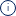MAI SUNT 00:00:00:00

MAI SUNT

X

# The Principia

## De (autor): Sir Isaac NewtonSir Isaac Newton's Principia Mathematica (Mathematical Principles) is considered to be among the finest scientific works ever published. His grand unifying idea of gravitation, with effects extending throughout the solar system, explains by one principle such diverse phenomena as the tides, the precession of the equinoxes, and the irregularities of the moon's motion. Newton's brilliant and revolutionary contributions to science explained the workings of a large part of inanimate nature mathematically and suggested that the remainder might be understood in a similar fashion. By taking known facts, forming a theory that explained them in mathematical terms, deducing consequences from the theory, and comparing the results with observed and experimental facts, Newton united, for the first time, the explication of physical phenomena with the means of prediction. By beginning with the physical axioms of the laws of motion and gravitation, he converted physics from a mere science of explanation into a general mathematical system.
Citeste mai mult

-15%

78.95Lei

92.88 Lei

Sau 7895 de puncteFiecare comanda noua reprezinta o investitie pentru viitoarele tale comenzi. Orice comanda plasata de pe un cont de utilizator primeste in schimb un numar de puncte de fidelitate, In conformitate cu regulile de conversiune stabilite. Punctele acumulate sunt incarcate automat in contul tau si pot fi folosite ulterior, pentru plata urmatoarelor comenzi.

Livrare in 3-5 saptamani

#### Descrierea produsului

Sir Isaac Newton's Principia Mathematica (Mathematical Principles) is considered to be among the finest scientific works ever published. His grand unifying idea of gravitation, with effects extending throughout the solar system, explains by one principle such diverse phenomena as the tides, the precession of the equinoxes, and the irregularities of the moon's motion. Newton's brilliant and revolutionary contributions to science explained the workings of a large part of inanimate nature mathematically and suggested that the remainder might be understood in a similar fashion. By taking known facts, forming a theory that explained them in mathematical terms, deducing consequences from the theory, and comparing the results with observed and experimental facts, Newton united, for the first time, the explication of physical phenomena with the means of prediction. By beginning with the physical axioms of the laws of motion and gravitation, he converted physics from a mere science of explanation into a general mathematical system.
Citeste mai mult

Detaliile produsului

## De pe acelasi raft

Noi suntem despre cărţi şi la fel este şi Newsletter-ul nostru

Abonează-te pentru a afla rapid care sunt promoţiile zilei, noutăţile şi recomandările noastre.